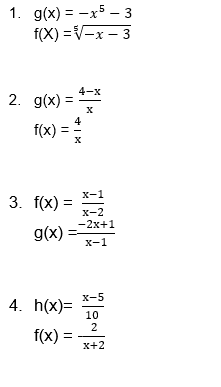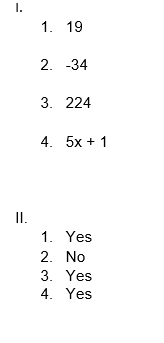# Composite and Inverse Functions Worksheet (with answers +PDF)

In mathematics, an inverse is a function that serves to “undo” another function. That is, if f(x)produces y, then putting y into the inverse of (f) produces the output x. A function (f) that has an inverse is called invertible and the inverse is denoted by f ^-1When two functions are combined in such a way that the output of one function becomes the input of another function, then this is referred to as a composite function.

## What is the “Composite and Inverse Functions Worksheet “with answers +PDF”?

This worksheet will explore some of the Composite and Inverse Functions.

## What are Composite and Inverse Functions?

The range of the given function becomes the domain of the inverse function, and the domain of the original function is represented with f^-1 with respect to the original function f. The composition of functions is the process of combining two or more functions into a single function. A function is an example of work. Take making bread as an example.

This worksheet will help you solve the Composite and Inverse Functions and also it provides a technique on how to solve them.

Instructions on how to use the “Composite and Inverse Functions Worksheet “with answers +PDF”

Study the concept and examples given and try to solve the given exercises below.

## Conclusion

Along with performing the calculation, let’s examine the mathematical function composition. Let’s look at how to determine its domain and range as well. Let’s find out more about the inverse function as well as how to find it.

If you have any inquiries or feedback, please let us know.

## Composite and Inverse Functions Worksheet

The Composition of Functions

The composition of the function f with g is denoted by f ◦ g and is defined by the equation

(f ◦g) (x) = f(g(x))

The domain of the composite function f ◦ g is the set of all x such that:

• x is in the domain of g and
• g(x) is in the domain of

Forming Composite Functions

Example 1

Given f(x) = 5x + 2 and g(x) = 3x − 4, find (f ◦ g) (x)and (g ◦ f) (x)

Inverse Function

Let f and g be two functions such that

• f(g(x)) = x for every x in the domain of g, and
• g(f(x)) = x for every x in the domain of f.

The function g is the inverse of the function f and is denoted by f^-1 (read “f-inverse”). Thus f (f^−1 (x) = x and f^−1 f(x)= x. The domain of f is equal to the range of f^−1 and vice versa.

Verifying Inverse Functions

Example 2:

Verify that each function is the inverse of the other:

f(x) = 6x and g(x) =x6

Composite Functions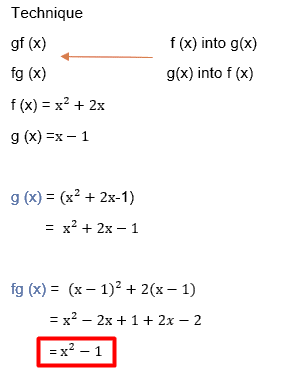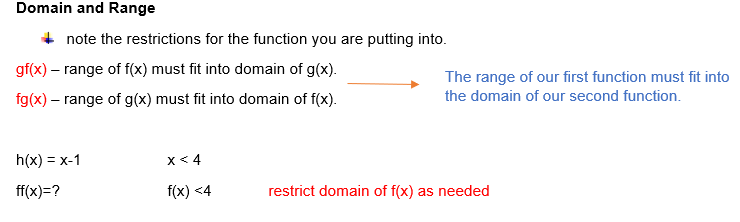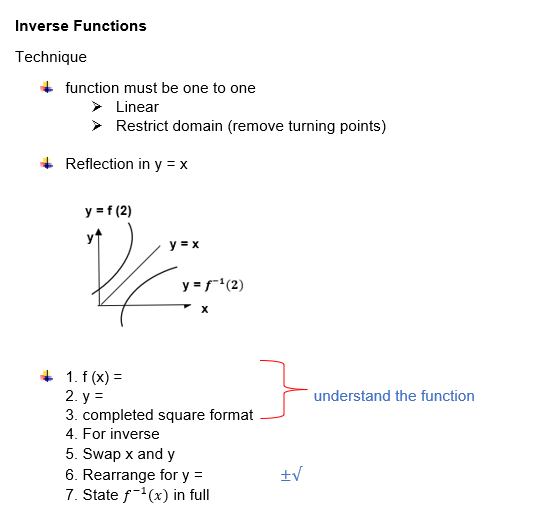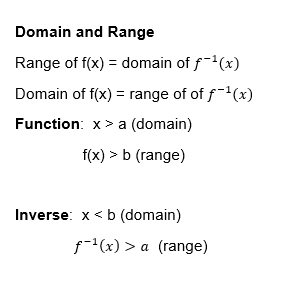Inverse One-to-one Functions

For example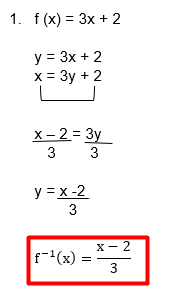Composition of Function (f ◦ g) (x) = f(g(x))

For example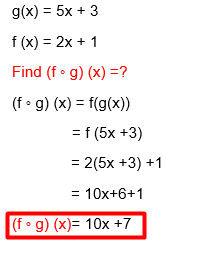## Worksheet

1. Composition of Functions

Using f(x) = 5x + 4 and g(x) = x – 3, find:

1.  f (g (6))
1. G (f (-7))
1. f (f (8))
1.  g(f(x))
1. Inverse Functions

State if the given functions are inverses.